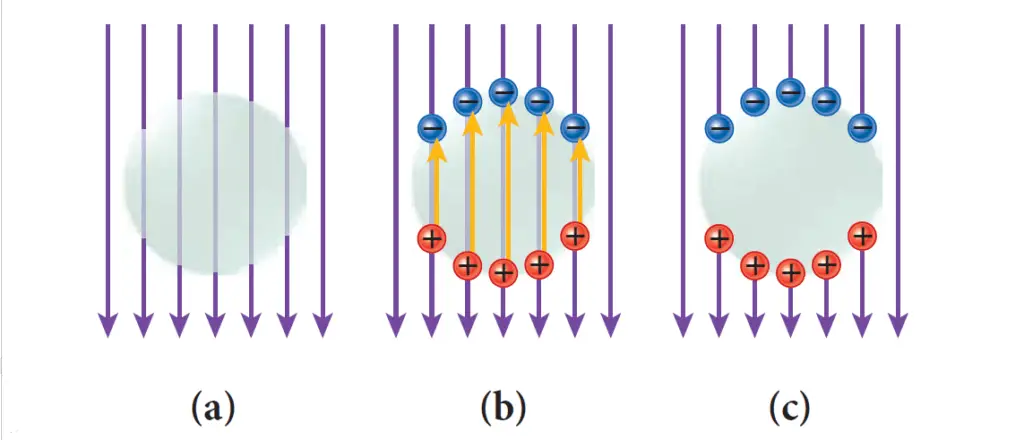# The electrostatic field inside any isolated conductor is always zero

Last updated on December 9th, 2022 at 08:33 am

The electrostatic field inside any isolated conductor is always zero. In a cavity in a conductor, the net charge and the electric field are always zero. Let’s see how we can say that.

Note that, these are important consequences of Gauss’s Law.

## The electrostatic field inside any isolated conductor is always zero

Let’s suppose a net electric field exists (actually it doesn’t exist, we will see that here) at some moment, at some point inside an isolated conductor; see Figure 1a. But every conductor has free electrons inside it (blue circles in Figure 1b), which can move rapidly in response to any net external electric field, leaving behind positively charged ions (red circles in Figure 1b).figure 1: Shielding of an external electric field (purple vertical arrows) from the inside of a conductor. The electrostatic field inside any isolated conductor is always zero

The charges will move to the outer surface of the conductor, leaving no net accumulation of charge inside the volume of the conductor. These charges will in turn create an electric field inside the conductor (yellow arrows in Figure 1b), and they will move around until the electric field produced by them exactly cancels the external electric field.

The net electric field thus becomes zero everywhere inside the conductor (Figure 1c).

## In a cavity in a conductor, the net charge and the electric field are always zero

If a cavity is scooped out of a conducting body, the net charge and thus the electric field inside this cavity is always zero, no matter how strongly the conductor is charged or how strong an external electric field acts on it.

To prove this, we assume a closed Gaussian surface surrounds the cavity, completely inside the conductor. From the preceding discussion (see Figure 1 above), we know that at each point of this surface (completely inside the conductor), the field is zero. Therefore, the net flux over this surface is also zero.

By Gauss’s Law, it then follows that this surface encloses zero net charges.

If there were equal amounts of positive and negative charges on the cavity surface (and thus no net charge), this charge would not be stationary, as the positive and negative charges would be attracted to each other and would be free to move around the cavity surface to cancel each other. Therefore, any cavity inside a conductor is totally shielded from any external electric field.

Thus in a cavity in a conductor, the net charge and the electric field are always zero.

## Electrostatic Shielding

Any cavity inside a conductor is totally shielded from any external electric field. This effect is sometimes called electrostatic shielding.

The conductor surrounding the cavity does not have to be a solid piece of metal; even a wire mesh is sufficient to provide shielding. Also, read Faraday Cage.

See also  The motion of a charged particle in an electric field between two parallel plates
Scroll to top
error: physicsTeacher.in